Mathematical Problems For the Next Century
Steve Smale

Department of Mathematics • City University of Hong Kong • Kowloon, Hong Kong • August

Introduction. V. I. Arnold, on behalf of the International Mathematical Union has written to a number of mathematicians with a suggestion that they describe some great problems for the next century. This report is my response. Arnold's invitation is inspired in part by Hilbert's list of 1900 (see e.g. (Browder, 1976)) and I have used that list to help design this essay. I have listed 18 problems, chosen with these criteria:

• Simple statement. Also preferably mathematically precise, and best even with a yes or no answer.
• Personal acquaintance with the problem. I have not found it easy.
• A belief that the question, its solution, partial results or even attempts at its solution are likely to have great importance for mathematics and its

Some of these problems are well known. In fact, included are what I believe to be the three greatest open problems of mathematics: the Riemann Hypothesis, Poincaré Conjecture, and"Does P=NP?" Besides the Riemann Hypothesis, one below is on Hilbert's list (Hilbert's 16th Problem). There is a certain overlap with my earlier paper "Dynamics retrospective, great problems, attempts that failed" (Smale, 1991).

Let us Begin

1 Lecture given on the occasion of Arnold's 60th birthday at the Fields Institute, Toronto, June 1997. Original version appeared in the Mathematical Intelligencer Vol 20, (Spring 1998) pp 7-15

Problem 1 • The Riemann Hypothesis

Are those zeros of the Riemann zeta function, dened by analytic continuation from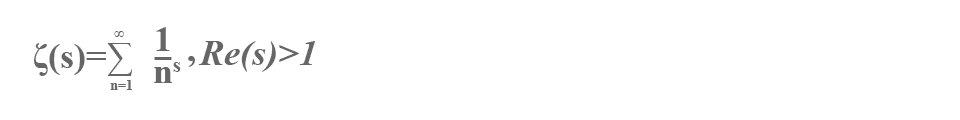This was problem #8 on Hilbert's list. There are many fine books on the zeta function and the Riemann hypothesis which are easy to locate. I leave the matter at this.

Problem 2 • The Poincare Conjecture

Suppose that a compact connected 3-dimensional manifold has the property that every circle in it can be deformed to a point. Then must it be homeomorphic to the 3-sphere?

The n-sphere is the spaceA compact n-dimensional manifold can be thought of as a closed bounded n-dimensional surface (di erentiable and non-singular) in some Euclidean space.

The n-dimensional Poincare conjecture asserts that a compact n-dimensional manifold M having the property that every map $f:{s}^{k}\to M$, $k (or equivalently, $k\le n/2$) can be deformed to a point, must be homeomorphic to ${s}^{n}$ .

Henri Poincaré studied these problems in his pioneering papers in topology. Poincare in 1900 (see Poincaré, 1953, pp 338{370) announced a proof of the general n-dimensional case. Subsequently (in 1904) he found a counter-example to his first version of the statement (Poincaré 1953, pp 435{498). In the second paper he limits himself to $n=3$, and states the 3-dimensional case as the problem above (not actually as a \conjecture").

My own relationship with this problem is described in the story (Smale, 1990a). There I wrote

I first heard of the Poincaré conjecture in 1955 in Ann Arbor at the time I was writing a thesis on a problem of topology. Just a short time later, I felt that I had found a proof (3 dimensions). Hans Samelson was in his office, and very excitedly I sketched my ideas to him. ... After leaving the office, I realized that my "proof" hadn't used any hypothesis on the 3-manifold.

In 1960, "on the beaches of Rio", I gave an armative answer to the n-dimensional Poincare conjecture for $n>4$. In 1982, Mike Freedman gave an affirmative answer for $n=4$. (Note: for $n>4$, I proved the stronger result that M was the smooth union of two balls, $M={D}^{n}\cup {D}^{n}$; that result is unproved for $n=4$, today.)

For background on these matters, besides the above references, see (Smale, 1963).

Many other mathematicians after Poincaré have claimed proofs of the 3-dimensional case. See (Taubes, 1987) for an account of some of these attempts.

A reason that Poincaré's conjecture is fundamental in the history of mathematics is that it helped give focus to a manifold as an object of study in its own right. In this way, Poincaré influenced much of 20th century mathematics with its attention to geometric objects including eventually algebraic varieties, Riemannian manifolds, etc.

I hold the conviction that there is a comparable phenomenon today in the notion of a "polynomial time algorithm". Algorithms are becoming worthy of analysis in their own right, not merely as a means to solve other problems. Thus I am suggesting that as the study of the set of solutions of an equation (e.g. a manifold) played such an important role in 20th century mathematics, the study of finding the solutions (e.g. an algorithm) may play an equally important role in the next century.

Problem 3 • Does P=NP?

I sometimes consider this problem as a gift to mathematics from computer science. It may be useful to put it into a form which looks more like traditional mathematics.

Towards this end first consider the Hilbert Nullstellensatz over the complex numbers. Thus let ${f}_{1}$ ,…, ${f}_{k}$ be complex polynomials in n variables; we are asked to decide if they have a common zero $\zeta \in {C}^{n}$. The Nullstellensatz asserts that this is not the case if and only if there are complex polynomials ${g}_{1}$,…, ${g}_{k}$ in n variables satisfying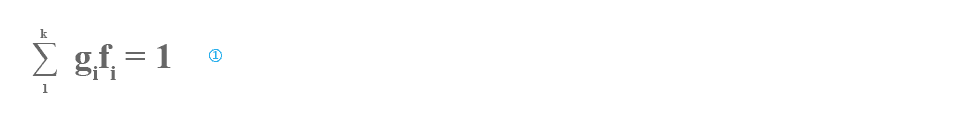as an identity of polynomials.

The effective Nullstellensatz as established by Brownawell (1987) and others, states that in , the degrees of the gi may be assumed to satisfy

$\mathrm{deg}{g}_{i}\le \mathrm{max}{\left(3,D\right)}^{n}$, $D=\mathrm{max}\mathrm{deg}{f}^{i}$.

With this degree bound the decidability problem becomes one of linear algebra. Given the coefficients of the ${f}_{i}$ one can check if has a solution whose unknowns are the coefficients of the ${g}_{i}$. Thus one has an algorithm to decide the Nullstellensatz. The number of arithmetic steps required grows exponentially in the number of coefficients of the ${f}_{i}$ (the input size).

Conjecture (over $C$ ). There is no polynomial time algorithm for deciding the Hilbert nullstellensatz over $C$ .

A polynomial time algorithm is one in which the number of arithmetic steps is bounded by a polynomial in the number of coefficients of the ${f}_{i}$, or in other words, is polynomially bounded.

To make mathematical sense of this conjecture, one has need of a formal definition of algorithm. In this context, the traditional definition of Turing machine makes no sense. In (Blum-Shub-Smale, 1989) a satisfactory satisfactory is proposed, and the associated theory is exposed in (Blum-Cucker-Shub-Smale (or BCSS), 1997).

Very briefly, a machine over $C$ has as inputs a nite string ( ... ${x}_{-1}$; ${x}_{0}$; ${x}_{1}$, ... ) of complex numbers and the same for states and outputs. Computations on states include arithmetic operations and shifts on the string. Finally, a branch operation on "${x}_{1}=0?$" is provided.

The size of an input is the number of elements in the input string. The time of a computation is the number of machine operations used in the passage from input to output. Thus a polynomial time algorithm over $C$ is well-defined.

Note that all that has been said about the machines and the conjecture use only the structure of $C$ as a field and hence both the machines and conjecture make sense over any field. In particular if the field is ${Z}_{2}$ of two elements, we have the Turing machines.

Consider the decision problem: Given (as input) $k$ polynomials in n variables with coefficients in ${Z}_{2}$. Decide if there is a common zero $\zeta \in {\left({Z}_{2}\right)}^{n}$?

This is a reformulation of the classic conjecture $P\ne NP$.

In the above we have bypassed the basic ideas and theorems related to NP-completeness. For the classic case of Cook and Karp, see (Garey-Johnson, 1979), and for the theory over an arbitrary field, see BCSS.

It is useful for some of the problems below (7,9,17,18) to have the definition of a machine over the real numbers $R$. In fact, the only change in the definition over $C$ , is to take the branch operation to be "${x}_{1}\le 0$?"

Remark: In his foreward to BCSS, Dick Karp writes that he is inclined to think that the complexity question over $C$ above and the classical P versus NP question are very different and need to be attacked independently. On the other hand just after our book went to press, I noticed that there was a strong connection between the old and the new theories. BPP denotes the set of problems that can be solved in polynomial time (classically) using randomization. It is in a certain practical sense, almost the same as the class P. By using a short argument, reduction of polynomial systems modulo a random prime, supported by a useful conversation with Manuel Blum, I saw that, not $NP\subset BPP$ (classic) implies $P\ne NP$ over $C$ , that is, the conjecture over $C$ above. This result is also implicit in (Cucker et al, 1995).

Problem 4 • Integer zeros of a polynomial of one variable

Let us start by defining a diophantine invariant $\tau$ motivated by complexity theory. A program for a polynomial $f\in Z\left[t\right]$ of one variable with integer coefficients is the object ($1$, $t$, ${u}_{1}$, ... , ${u}_{k}$) where ${u}_{k}=f$, and for all $l$, ${u}_{l}={u}_{i}\circ {u}_{j}$ , $i,j, and$\circ$ is $+$ or $-$ or $×$. Here ${u}_{0}=t$, ${u}_{-1}=1$. Then $\tau \left(f\right)$ is the minimum of $k$ over all such programs.

Is the number of distinct integer zeros of $f$, polynomially bounded by $\tau \left(f\right)$?

In other words, isHere $Z\left(f\right)$ is the number of distinct integer zeros of $f$ with $a$, $c$ universal constants.

From earlier results of Strassen, communicated via Sch¨onhage, Shub, and B¨urgisser, it follows that the exponent c has to be at least 2.

Mike Shub and I discovered this problem in our complexity studies. We proved that an affirmative answer implied the intractibility of the Nullstellensatz as a decision problem over $C$ and thus $P\ne NP$ over $C$ . See (Shub-Smale, 1995) and also BCSS.

Since the degree of $f$ is less than or equal to ${2}^{\tau }$ , $\tau =\tau \left(f\right)$, there are no more than ${2}^{\tau }$ zeros altogether.

For Chebyshev polynomials, the number of distinct real zeros grows exponentially with $\tau$ .

Many of the classic diophantine problems are in two or more variables. This problem asks for an estimate in just one variable, and nevertheless seems not so easy.

Here is a related problem. A program for an integer m is the object ($1$;${m}_{1}$, ... ,${m}_{l}$ ) where ${m}_{l}=m$, ${m}_{0}=1$, ${m}_{q}={m}_{o}\circ {m}_{j}$, $i,j and $\circ$ = $+$; $-$ or $×$. Then let $\tau \left(m\right)$ be the minimum of $l$, over all such programs. Thus $\tau \left(m\right)$ represents the shortest way to build up an integer $m$ starting from 1 using plus, minus, and times.

Problem: Is there a constant $c$ such that $\tau \left(k!\right)\le {\left(\mathrm{log}k\right)}^{c}$ for all integers $k$? One might expect this to be false, so that $k!$ is "hard to compute" (see Shub-Smale, 1995).

Problem 5 • Height bounds for diophantine curves

Can one decide if a diophantine equation $f\left(x,y\right)=0$ (input $f\in Z\left[u,v\right]$) has an integer solution, $\left(x,y\right)$, in time ${2}^{s}{}^{c}$ where $c$ is a universal constant? That is, can the problem be decided in exponential time?

Here $s=s\left(f\right)$ is the size of $f$ defined by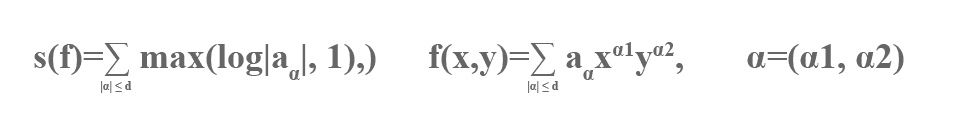and $\left|\alpha \right|={\alpha }_{1}+{\alpha }_{2}$, ${\alpha }_{i}\ge 0$.

The Turing model of computation is supposed, so “time”is the number of Turing operations.

This problem is essentially posed in (Cucker-Koiran-Smale, 1997), but it is a version of a well-known problem in number theory. The size $s\left(f\right)$ is a version of the “height" of $f$. Our problem is likely to be very dicult since it is not even known if one can decide this diophantine problem at all, let alone in exponential time. The solution of Hilbert's tenth problem by Matiyasevich (see Matiyasevich, 1993), using work of Davis, Robinson, Putnam shows the undecidability if the number of variables (27 is sucient if not 20 or even 11) is not restricted. A remaining important unsolved problem in this connection is:

Can one decide if there is a rational number solution to a given diophantine equation (any number of variables)?

The computer science notion of $NP$ is relevant to our main problem above. A problem in $NP$ is seen to be solvable in exponential time. The simple standard argument is given by noting that the test, evaluation of a polynomial, is done in polynomial time. Thus one might well ask the stronger question; is the two variable diophantine problem in $NP$?

To simplify the discussion we will now assume that the genus of the curve (defined by) $f$ is positive. The genus of a non-singular curve is the number of ”handles” in the homogenized curve of complex zeros. For a singular curve one can de ne the genus by taking an appropriate associated non-singular curve.

Consider the following hypothesis.

Height bound hypothesis: If the curve $f$, of positive genus, has any integer solution, then it has a solution $\left(a,b\right)$ satisfying the estimate; $\mathrm{log}\mathrm{max}\left(\left|a\right|,\left|b\right|\right)$ is polynomially bounded by $s\left(f\right)$.

For curves of positive genus, the height bound hypothesis implies that such a class of diophantine equations is in $NP$, and hence answers our main problem affirmatively.

One may ask how the height bound hypothesis relates to older conjectures in number theory. Thus let the strong height bound hypothesis be the strengthening of the height bound hypothesis to include all integer solutions. The Lang-Stark conjecture (see Lang, 1991), on certain curves of genus one, is implied by the strong height bound hypothesis, if one replaces our polynomially bounded by linearly bounded.

We don't claim real evidence for the height bound hypothesis. However there is in the background everywhere in this section, the Siegel theorem that there are only finitely many integer points on any diophantine curve of positive genus. A great challenge is to make Siegel's theorem effective; the height bound hypothesis is a version of such a goal.

For the case of genus one, and genus zero in case of a finite number of zeros, one does have the effectiveness results of Baker, and Baker-Coates, (see Baker, 1979), but even here they are somewhat weaker than the estimate of the strong height bound hypothesis.

The height bound hypothesis is false without the condition on the genus. This follows from the fact that the smallest integer solution of the "non-Pellian" equation ${x}^{2}-d{y}^{2}=-1$ can be very large relative to a family of $d$ (see Lagarias 1980). (Mazur (1994) points out a similar phenomenon for Pell's equation, conditional on the Gauss class number conjecture). Lagarias (1979) proves none the less that this equation (ie. the feasibility) is in $NP$ and moreover that the set of general binary quadratic equations is in $NP$. Manders-Adleman (1978) have shown some $NP$-completeness results on these problems.

There is also the more difficult version of all these problems when one uses the sparse representation of $f$, ie., in the de nition of $s\left(f\right)$ above delete the terms in which ${a}_{\alpha }=0$. Even the one variable case while true is not immediate. See (Cucker-Koiran-Smale, 1997), and (Lenstra, 1997).

Problem 6 • Finiteness of the number of relative equilibria in celestial mechanics

Is the number of relative equilibria nite, in the n-body problem of celestial mechanics, for any choice of positive real numbers ${m}_{1}$, ... , ${m}_{n}$ as the masses?

The problem is in Wintner's book (1941) on celestial mechanics. A relative equilibrium is a solution to Newton's equations which is induced by a plane rotation.

For the 3-body problem there are five relative equilibria: three found by Lagrange, two by Euler. There are “the Trojans” in the solar system, which correspond to the Lagrange relative equilibria. For 4-bodies the finiteness is unknown.

In (Smale, 1970), I interpreted the relative equilibria as critical points of a function induced by the potential of the planar $n$-body problem. More precisely the relative equilibria correspond to the critical points ofThe rotation group $SO\left(2\right)$ acts on $S-\Delta$ and is induced on the quotient from the potential function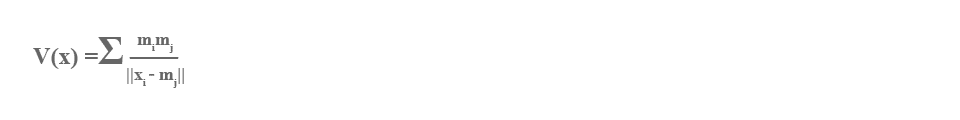Note that $V:S\to R$ is invariant under the rotation group $SO\left(2\right)$ and that the quotient space $S/SO\left(2\right)$ is homeomorphic to complex projective space of dimension $n-2$.

Thus the question has the equivalent form:

For any choice of ${m}_{1}$, ... , ${m}_{n}$ , does of $\left(2\right)$ have a finite number of critical points?

Mike Shub (1970) has shown that the set of critical points is compact.

Say that (${m}_{1}$ , ... , ${m}_{n}$ ) is critical if the corresponding has a degenerate critical point.

What is the nature of the set of critical masses in the n-dimensional spaces of masses? Does it have measure zero? or nite Betti numbers?

Palmore (1976) has shown that it is empty in case $n=3$ and not empty in case $n=4$. Kuz'mina, Moeckel, Xia, Albouy, and McCord, (see e.g. McCord, 1996), have further results on these problems.

G. D. Birkhoff asked the question: what is the topology of the constant angular momentum submanifolds of the $n$ body problem? In (Smale, 1970) I solved this problem for the case of $n$ bodies in the plane. See also (Easton, 1971). The case for n bodies in 3-dimensional space remains open: one obstacle is the solution of Wintner's problem above. However, recently, McCord-Meyer-Wang (1998), solved Birkhoff's problem for the 3 body problem in 3-space. See this paper also for a good historical and mathematical background for many of these things.

Further background may be found in (Abraham-Marsden, 1978).

Problem 7 • Distribution of points on the 2-sphere.

Let ${V}_{n}\left(x\right)={\Sigma }_{1\le i where $x=\left({x}_{1},...,{x}_{n}\right)$, the ${x}_{i}$ are distinct points on the 2-sphere ${S}^{2}\subset {R}^{3}$ , and $\left|\left|{x}_{i}-{x}_{j}\right|\right|$ is the distance in ${R}^{3}$. Denote $mi{n}_{x}{V}_{N}\left(x\right)$ by ${V}_{N}$

Can one find (x1 ,... , xN ) such thatFor a precise version one could ask for a real number algorithm in the sense of BCSS which on input $N$ produces as output distinct ${x}_{1},...,{x}_{n}$ on the 2-sphere satisfying with halting time polynomial in $N$.

This problem emerged from complexity theory, jointly with Mike Shub (see Shub-Smale, 1993). It is motivated by finding a good starting polynomial for a homotopy algorithm for realizing the Fundamental Theorem of Algebra.

An $\left({x}_{1},...,{x}_{n}\right)=x$ such that ${V}_{N}\left(x\right)={V}_{N}$ is called an $N$-tuple of elliptic Fekete points (see Tsuji, 1959).

The function ${V}_{N}$ as a function of $N$ satisfiesIt is natural also to consider the functions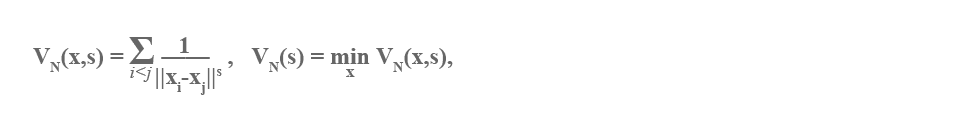$x$ as before and $0. The original $\left({x}_{1},...,{x}_{n}\right)=x$; ${V}_{N}$ correspond in a natural way to $s=0$, and for $s=1$, ${V}_{N}\left(x,1\right)$ is the Coulomb potential, and ${V}_{N}\left(1\right)$ corresponds to an equilibrium position of $N$ electrons constrained to lie on the two-sphere. There are similar problems for various $s$. One might equally well consider higher-dimensional spheres.

I had asked Ed Saff for some help in dealing with the main problem above. Subsequently, he and his colleagues produced a number of fine papers dealing with the subject and its ramifications. See (Kuijlaars-Saff, 1997) and (Saff-Kuijlaars, 1997) for background and further references. In Rakhmanov-Saff-Zhou (1994), one can find an algorithm where numerical evidence is provided to support ③ for $N\le 12,000$, with $c=114$.However, as was written in (Shub-Smale, 1993), “ ... this may not be so easy since there are saddle points of index $N$ (on a great circle in ${S}^{2}$, evenly spaced $N$ points, ${x}_{1}$ , ... , ${x}_{N}$). Also the various symmetries that ${W}_{N}$ possesses will confuse the picture."

Problem 8 • Introduction of dynamics into economic theory

The following problem is not one of pure mathematics, but lies on the interface of economics and mathematics. It has been solved only in quite limited situations.

Extend the mathematical model of general equilibrium theory to include price adjustments.

There is a (static) theory of equilibrium prices in economics starting with Walras and firmly grounded in the work of Arrow and Debreu (see Debreu, 1959). For the simplest case of one market this amounts to the equation"supply equals demand" and a natural dynamics is easily found (Samuelson, 1971). For several markets, the situation is complex.

There is a function called the excess demand, $Z\left(P\right)=D\left(P\right)-S\left(P\right)$ from the space of prices to the space of commodities. Both the demand $D$ and supply $S$ are de ned by aggregation over the individual agents. Economics justi es conditions on individual behavior which lead to axioms on $Z$. These axioms for the excess demand map $Z:{R}_{+}^{l}\to {R}^{l}$ are:By $\left(1\right)$, $\left(2\right)$, $\left(3\right)$, $Z$ may be interpreted as a vector field on the intersection of the $\left(l-1\right)$-sphere with the positive orthant, pointing inward on the boundary. The existence of an equilibrium price vector ${p}^{*}$ follows from Hopf's theorem, so that $Z\left({p}^{*}\right)=0$, and "supplyequals demand".

Problem 8 asks for a dynamical model, whose states are price vectors (perhaps enlarged to include other economic variables). This theory should be compatible with the existing equilibrium theory. A most desirable feature is to have the time development of prices determined by the individual actions of economic agents.

I worked on this problem for several years, feeling that it was the main problem of economic theory (Smale, 1976). See also (Smale, 1981a) for background.

Problem 9: The linear programming problem.

Is there a polynomial time algorithm over the real numbers which decides the feasibility of the linear system of inequalities ${A}_{x}\ge b$?

The algorithm requested by this problem is one given by a real number machine in the sense of BCSS (see also Problem 3). The system ${A}_{x}\ge b$ has as input an $m×n$ real matrix $A$ and a vector $b\in {R}^{m}$ and the problem asks, is there some $x\in {R}^{n}$ with ${\Sigma }_{j=1}^{n}{a}_{ij}{x}_{j}\ge {b}_{i}$ for all $i=1$,...,$m$? Time is measured by the number of arithmetic operations. This problem is in BCSS, page 275.

This is a decision version of the optimization problem of linear programming. Given $A$ , $b$ as above and $c\in {R}^{n}$ decide if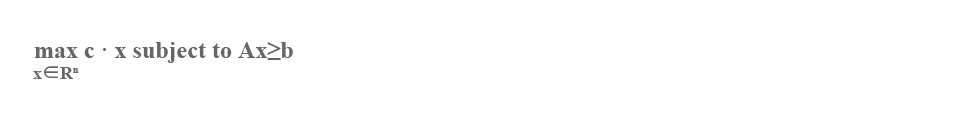exists, and if so, output such an $x$.

The famous simplex method of Dantzig provides an algorithm for both problems (over $R$ )but Klee and Minty showed that it was exponentially slow in the worst case. On the other hand, Borgwardt, and I, each approach with subsequent important support from Haimovich,showed that it was polynomial time on the average. For all of these things, see (Schrijver,1986).

In terms of the Turing model of computation, using rational numbers, $Q$ , and cost measured by "bits", there is a parallel development. Starting with ideas of Yudin-Nemirovsky,Khachian found a polynomial time algorithm (the ellipsoid method) for the linear programming problem. Subsequently Karmarkar with his \interior point method" found a practical algorithm for this problem, which he showed ran in polynomial time in the Turing model. For all these things one can see (Grötschel-Lovász-Schrijver, 1993) as well as (Schrijver, 1986).

Closer to the main problem above over $R$ is a similar problem asking for a "strongly polynomial algorithm" for solving these linear programming problems. This is an algorithm over $Q$ which is polynomial time in the sense of BCSS and moreover is polynomial time in the Turing sense. Partial results are due to Megiddo and especially Tardos (see (Grötschel-Lovász-Schrijver, 1993)).

For the problem over $R$ there are also references (Barvinok-Vershik, 1993) and (Traub-Wo´zniakowski, 1982).

It is my belief that this problem number nine is the main unsolved problem of linearprogramming theory. The use of real number algorithms plays a natural role in a subject where the most important measure of cost is the number of arithmetic operations. See Problem 3; see also Chapter 1 of BCSS where the use of our real number model in the theory of computation is argued.

The ability of real number machines to deal with round-off error is investigated in (Cucker-Smale, 1997).

Problem 10: The Closing Lemma.

Let p be a non-wandering point of a di eomorphism $S:M\to M$ of a compact manifold. Can $S$ be arbitrarily well approximated with derivatives of order r(${C}^{r}$ approximation) for each r, by $T:M\to M$ so that p is a periodic point of $T$?

A non-wandering point $p\in M$ is one with the property that for each neighborhood $U$ of $p$ there is a $k\in Z$ such that ${S}^{k}U\cap U\ne \phi$. Here ${S}^{k}$ is the $k$th iterate of $S$. Moreover $p$ is a periodic point of period $m$ if ${T}^{m}\left(p\right)=p$.

This is the discrete form of the famous"closing lemma" which in the ${C}^{1}$ case has been solved affrmatively by Charles Pugh (1967).

There is an easy ${C}^{0}$ approximation with the desired property. Peixoto observed that this argument failed for ${C}^{1}$ approximations correcting a mistake of René Thom (René told me that this was his biggest mistake).

Pugh-Robinson (1983) proved the closing lemma with ${C}^{1}$ approximations for the Hamiltonian version. Peixoto gave an affrmative answer with ${C}^{r}$ approximations, any $r$, for the circle. Recently the closing lemma has been given additional importance by the work of Hayashi (1997); see also (Wen-Xia, 1997).

Problem 11: Is one-dimensional dynamics generally hyperbolic?

Can a complex polynomial $T$ be approximated by one of the same degree with the property that every critical point tends to a periodic sink under iteration?

This is unsolved even for polynomials of degree 2. Here a polynomial map $T:C\to C$ ($C$ the complex numbers) is considered a discrete dynamical system by iteration. So if $z\in C$ ,its orbit in time, $z={z}_{0},{z}_{1},{z}_{2},$...is defined by ${z}_{i}=T\left({z}_{i-1}\right)$ and $i$ may be interpreted as time (discrete). A fixed point w of $T$, $\left(T\left(w\right)=w\right)$ is a sink if the derivative $T\text{'}\left(w\right)$ of $T$ at $w$ has absolute value less than 1. A periodic sink of $T$ of period p is a sink for ${T}^{p}$. A critical point of $T$ is just a point where the derivative of $T$ is zero.

While the problem is now made precise it is useful to see it in the framework of hyperbolic dynamics from the 1960's.

A fixed point $x$ of a diffeomorphism $T:M\to M$ is hyperbolic if the derivative $DT\left(x\right)$ of $T$ at $x$ (as a linear automorphism of the tangent space) has no eigenvalue of absolute value 1. If $x$ is a periodic point of period $p$, then $x$ is hyperbolic if it is a hyperbolic xed point of ${T}^{p}$. The notion of hyperbolic extends naturally to $\Omega$, the closure of the set of non-wandering points (see Problem 10).

A dynamical system $T\in$Diff$\left(M\right)$ is called hyperbolic (or satisfies Axiom A) if the periodic points are dense $\Omega$ in $\Omega$ and is hyperbolic (see Smale, 1967 or 1980). We assume also a no cycle condition. The work of many people, especially Ricardo Mañé, has identified hyperbolic dynamics with a strong notion of the stability of the dynamics called structural stability. There is even the beginning of a structure theory for this class of dynamics.

While hyperbolic systems constitute a large set of dynamics, an even larger set, including applied chaotic dynamics, lies beyond. The concept of hyperbolicity extends from the invertible dynamics to the case of our problem above, polynomial maps from $C$ to $C$ . Classical complex variable theory permits recasting the problem to an equivalent one:

Can a polynomial map $T:C\to C$ be approximated by one which is hyperbolic?

The theory of complex one-dimensional dynamics was begun by Fatou and Julia towards the beginning of this century. In the 1960's I asked my thesis student John Guckenheimer to look at this literature and try to solve the above problem (among other things). His thesis (see Chern-Smale, 1970) contains the affirmative answer, but with a gap in the proof. Now the problem stands open as the fundamental problem of one-dimensional dynamics.

Complex 1-dimensional dynamics has become a fluorishing subject and includes important contributions of Douady-Hubbard, Sullivan, Yoccoz, McMullen, among many others. See (McMullen, 1994).

There is a parallel field of real 1-dimensional dynamics of a smooth map $T:I\to I$,$I=\left[0,1\right]$.

Problem: Can a smooth map $T:\left[0,1\right]\to \left[0,1\right]$ be ${C}^{r}$ approximated by one which is hyperbolic, for all $r>1$?

About the time of Guckenheimer's thesis, I asked Ziggy Nitecki to study this problem.My earlier negligence was compounded in not catching the mistake in Nitecki's thesis (see Chern-Smale, 1970), which purported to give an armative proof.

Subsequently, Jakobson (1971) answered the problem for ${C}^{1}$ approximations, but the general case remains open. See de Melo-van Strien (1993) for background.

More recently there is the work of Lyubich (1997), and of Graczyk-Swiatek (1997), which gives a positive solution for the real case when $T$ is a quadratic map.

Let me remark on the mistakes in the published theses of my students, Guckenheimer and Nitecki mentioned above, Thom's mistake referred to in Problem 10 and Poincaré's mistake in the Poincaré conjecture of Problem 2. Mistakes happen frequently in published mathematics; I certainly have made my share. Especially in the early development of a subject, one is likely to err. Here oftentimes not only are the main concepts confused, but even the definitions are ambiguous. Thus there is the need to heed well the establishment of good foundations for a new subject. These considerations are a motivating factor in my efforts to help create a more solid foundations for numerical analysis (as in BCSS or Smale,1990b).

Let me point out a story about Poincaré as told by Diacu-Holmes (1996) or Barrow-Green(1996). When Poincaré discovered a mistake in his theory of celestial mechanics, he had the copies of Acta Mathematica in which his article appeared, destroyed; but at the same time he discovered a phenomenon in dynamics which is now called "chaos". About 60 years later,oblivious of these events, I foolishly hypothesized (see Smale, 1998) that chaos did not exist in dynamics!

Problem 12: Centralizers of diffeomorphisms.

Can a diffeomorphism of a compact manifold $M$ onto itself be ${C}^{r}$ approximated,all $r\ge 1$, by one $T:M\to M$ which commutes with only its iterates?

Thus the centralizer of $T$ in the group of diffeomorphisms, Diff$\left(M\right)$ should be $\left\{{T}^{k}\mid k\in Z\right\}$.

I had started thinking about the centralizer in (Smale, 1963), but it was after Nancy Kopell's thesis with me (see Chern-Smale, 1970), answering the question affrmatively in case dim $M=1$, that I proposed this problem (Smale, 1967). Today it remains unsolved even for the 2-sphere.

One may also ask if the set of di eomorphisms of $M$ with trivial centralizer is dense and open in Diff$\left(M\right)$ with the ${C}^{r}$ topology.

The main work on these problems has been done by Palis-Yoccoz (1989), with almost complete answers in the case of hyperbolic dynamics (see Problem 11) for any manifold.

I wrote in (Smale, 1991), "I find this problem interesting in that it gives some focus in the dark realm, beyond hyperbolicity, where even the problems are hard to pose clearly."

Problem 13: Hilbert's 16th Problem.

Consider the di erential equation in ${R}^{2}$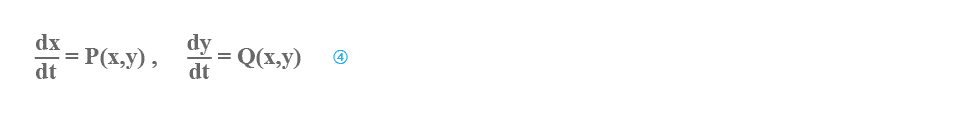where $P$ and $Q$ are polynomials. Is there a bound $K$ on the number of limit cycles of the form $K\le {d}^{q}$ where d is the maximum of the degrees of $P$ and $Q$, and $q$ is a universal constant?

This is a modern version of the second half of Hilbert's sixteenth problem. Except for the Riemann hypothesis, it seems to be the most elusive of Hilbert's problems.

In fact, since a paper of Petrovskii and Landis (1957) purporting to give a positive solution, the progress seems to be backwards. Earlier, Dulac (1923) claimed that the system(4) always has a finite number of limit cycles. After a gap in Petrovskii-Landis was found(see Petrovskii-Landis, 1959), Ilyashenko (1985) found an error in Dulac's paper. Moreover Shi Songling (1982) found a counter-example to the specific bounds of Petrovskii-Landis for the case $d=2$. Subsequently, two long works have appeared, independently, giving proofs of Dulac's assertion (Écalle, 1992) and (Ilyashenko, 1991). These two papers have yet to be thoroughly digested by the mathematical community.

Thus one has the finiteness, but no bounds. We will consider a special class where the finiteness is simple, but the bounds remain unproved.

The following corresponds to Lienard's equation (see e.g. (Hirsch-Smale, 1974))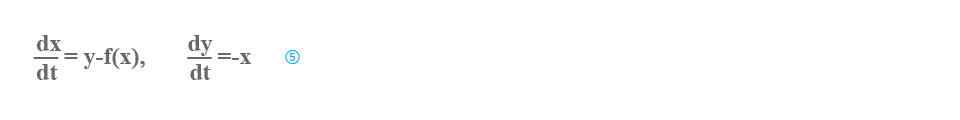where $f\left(x\right)$ is a real polynomial with leading term ${x}^{2k+1}$ and satisfying $f\left(0\right)=0$.

If $f\left(x\right)={x}^{3}-x$ then (5) is van der Pol's equation with one limit cycle. More generally,it can be easily shown that all the solutions of (5) circle around the unique equilibrium at$\left(0,0\right)$ in a clockwise direction. By following these curves, one defines a"Poincaré section",$T:{R}^{+}\to {R}^{+}$ where ${R}^{+}$ is the positive $y$ axis. The limit cycles of (5) are precisely the fixed points of $T$. In various talks I raised the question of estimating the number of these fixed points (via some new kind of xed point theorem?). In response, Linz, de Melo and Pugh(1977) found examples with $k$ different limit cycles and conjectured this number $k$ for the upper bound. Still no upper bound of the form ${\left(\mathrm{deg}f\right)}^{q}$ has been found. Since $T$ is analytic,it follows that (5) has a finite number of limit cycles for each $f$.

Moreover, I spoke on what I called the "Pugh problem" having learned of it from Charles Pugh. Consider the 1-variable differential equation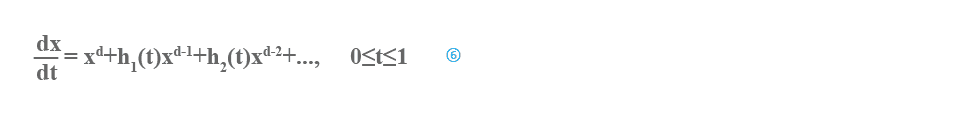where the ${h}_{i}$ are ${C}^{\infty }$ functions. Pugh had asked for bounds on $K\left(d\right)$ where $K\left(d\right)$ is the number of solutions satisfying the boundary condition $x\left(0\right)=x\left(1\right)$. Subsequently Curt McMullen has given an answer, sketched as follows.

For $d=0,1,2,$ the corresponding map is a translation, affine, Möbius respectively. For $d>2$ there can be arbitrarily many fixed points. To see this, notice that the closure of the space of mappings is a group. There are no closed subgroups lying between Möbius and the whole group of diffeomorphisms. More explicitly, if we start with polynomials of degree $>2$ and take the Lie algebra they generate, it is dense among continuous vector fields on the real line (in the topology of uniform convergence on compact sets.)

For more background see (Browder, 1976), (Ilyashenko-Yakovenko, 1995), (Lloyd and Lynch, 1988), and (Smale, 1991).

Problem 14: Lorenz attractor.

Is the dynamics of the ordinary differential equations of Lorenz (1963), that of the geometric Lorenz attractor of Williams, Guckenheimer and Yorke?

The Lorenz equations are: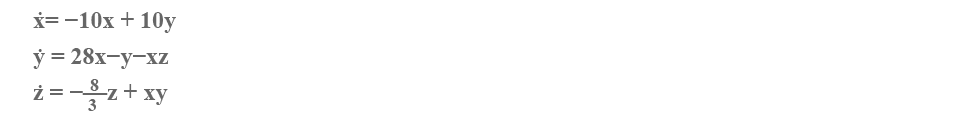Lorenz analysed by computer these equations to nd that most solutions tended to a certain attracting set, and in so doing, he produced an important early example of "chaos".

However mathematical proofs were lacking. This numerical work inspired the rigorous mathematical development of a geometrically defined ordinary differential equation which seems to have the same behavior (Yorke, Williams (1979), Guckenheimer-Williams (1979)). This geometric attractor has been analysed in detail and proved to be chaotic. Problem 14 asks if the dynamics of the original equations is the same as that of the geometric model. The most complete positive answer would be to describe a homeomorphism of ${R}^{3}$ to ${R}^{3}$ which would take solutions of the Lorenz equations to solutions of the geometric attractor. Actually the geometric Lorenz attractor is a two parameter family of dynamical systems and we are speaking of a member of this family.

An answer to this problem would be a step in establishing foundations for the eld of applied chaotic dynamical systems. Up to the present time, in the equations of engineering and physics, chaos has only been established in a weaker sense, that of proving the existence of horseshoes (e.g. Melnikov, Marsden and Holmes; see Guckenheimer and Holmes (1990)).

One problem is a paradoxical situation which occurs from the accumulation of roundo off error. While using the machine to study solutions of chaotic differential equations, the round-off error increases exponentially in time, by a fundamental property of chaos! The shadowing lemma of Anosov-Bowen has been extended to help deal with that paradox by Hammel-Yorke-Grebogi, Coomes-Kocak-Palmer (1996) and others.

Geometric, structurally stable, chaotic attractors in dynamics are in my paper (Smale(1967)). But these did not arise from any physical system.Some partial results on problem 14 are due to Rychlik and Robinson, see Robinson (1989).

Another related problem is: can one decide if a given dynamical system is chaotic? Is there an algorithm which, with coecients of a dynamical system as input, outputs yes if the dynamics is chaotic and no otherwise. For precision one could say that a dynamics is chaotic exactly when it contains a horseshoe.

Consider first the Turing machine point of view. Matiaysevich (1993) has described a polynomial over $Z$, $P\left(u,{x}_{1},...{x}_{n}\right)$, $n=27$, with the property that it can not be decided on input $u$ in $Z$ whether there is a zero $\left({x}_{1},...{x}_{n}\right)$ in ${Z}^{n}$. Richardson and Costa-Doria (1991)made a study of the function: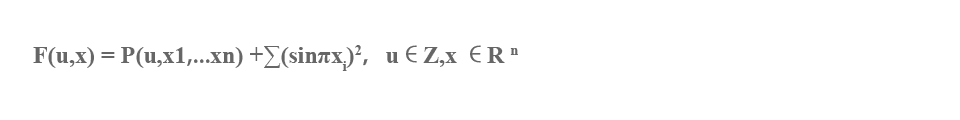Observe that one cannot decide the question: on input $u$, does this function have a zero in ${R}^{n}$. If one can't decide the existence of a zero, one can hardly expect to decide the existence of chaos. In fact Costa-Doria prove just that, chaos is undecidable.

However one could well object in a number of ways; this analysis is far from the standard and useful models of dynamics. For one thing, Turing machines would seem to be a poor idealization of algorithms used in this subject as argued in our manifesto (see Chapter one of BCSS).

Another approach is the way L. Blum and I (1992) dealt with the question of Penrose (1991), "is the Mandelbrot set decidable?". We made the question precise by putting it into the framework of machines over $R$ and then answered it in the negative.

The Mandelbrot set is a certain set of complex numbers which might be interpreted as having a high level of chaos (in fact having a one dimensional chaotic set) for a corresponding dynamics. Thus my result with Blum asserts that one can't decide if a given dynamics has a high level of chaos. It suggests an alternate route to Costa-Doria in questions of generally deciding if a given dynamics is chaotic.

There are many nuances here, related to properties of approximation, random algorithms, polynomial time, etc. See Problem 18 for further thoughts.

Problem 15: Navier-Stokes equations.

Do the Navier-Stokes equations on a 3-dimensional domain $\Omega$ in ${R}^{3}$ have a unique smooth solution for all time?

This is perhaps the most celebrated problem in partial differential equations. Let us be a little more precise. The Navier-Stokes equations may be writtenwhere a ${C}^{\infty }$ map $u:{R}_{+}×\Omega \to {R}^{3}$ and $p:\Omega \to R$ are to be found satisfying these equations, $u$ prescribed at $t=0$ and on the boundary $\partial \Omega$. Here ${R}_{+}=\left[0,\infty \right)$,and is the operator ${\Sigma }_{1}^{3}{u}_{i}\genfrac{}{}{0.1ex}{}{\partial }{{\partial }_{{x}_{i}}}$ ,and $v$ is a positive constant. See e.g. (Chorin-Marsden, 1993) for details.

Many mathematicians have contributed toward the understanding of this problem. An affirmative answer has been given in dimension 2 and in dimension 3 for $t$ in a small interval $\left[0,T\right]$. See (Temam, 1979) for background.

The solution of this problem might well be a fundamental step toward the very big problem of understanding turbulence. For example it could help realize the ideas of Ruelle-Takens (1971), which put the notion of a chaotic attractor into a model of turbulence. Seealso (Chorin-Marsden-Smale, 1977).

In (Smale, 1991) I asked if the solutions of the 2-dimensional Navier-Stokes equation with a forcing term on a torus must converge to an equilibrium as time tends to in nity. Babik-Vishik (1983) had given some evidence to the contrary. Subsequently Liu (1992) provided examples to show convergence to a more complicated attractor.

Problem 16: The Jacobian Conjecture.

Suppose $f:{C}^{n}\to {C}^{n}$ is a polynomial map with the property that the derivative at each point is non-singular. Then must $f$ be one to one?

Here ${C}^{n}$ is an n-dimensional complex Cartesian space, $f\left(z\right)=\left({f}_{1}\left(z\right),...,{f}_{n}\left(z\right)\right)$ and each ${f}_{i}$ is a polynomial in n variables. The derivative of $f$ at $z$, $Df\left(z\right):{C}^{n}\to {C}^{n}$ may be thought of as the matrix of partial derivatives and the non-singularity condition as Det $Df\left(z\right)\ne 0$.

If f is indeed injective then it is surjective and has an inverse which is a polynomial map.

The problem goes back to the 1930's and one can see the excellent surveys (Bass-Connell-Wright, 1982) and (van den Essen, 1997) for the importance, background, and related results.

Problem 17: Solving polynomial equations.

Can a zero of n complex polynomial equations in n unknowns be found approxi-mately, on the average, in polynomial time with a uniform algorithm?

More broadly, what are the features that distinguish the tractable from the intractable in the realm of solving polynomial systems of equations?

The nal theorem in the ve paper series, jointly done with Mike Shub, Bez I − V (see Shub-Smale, 1994) is exactly the italicized result without \uniform".

We review the de nitions. Consider $f:{C}^{n}\to {C}^{n}$, $f\left(z\right)=\left({f}_{1}\left(z\right),...,{f}_{n}\left(z\right)\right)$, $z=\left({z}_{1},...,{z}_{n}\right)$ where each ${f}_{i}$ is a polynomial in $n$ variables of degree ${d}_{i}$.

It is reasonable to make the ${f}_{i}$ into homogeneous polynomials by adding a new variable ${z}_{0}$, work in the corresponding projective space, and then translate the algorithm and results back to the initial ane problem.

Approximately can be de ned intrinsically using Newton's method and is necessary because of the classic results of Abel and Galois. Time is measured by the number of arithmetic operations and comparisons, using real machines (as in Problem 3) if one wants to be formal.

A probability measure must be put on the space of all such $f$, for each $d=\left({d}_{1},...,{d}_{n}\right)$ and the time of an algorithm is averaged over the space of $f$. Is there such an algorithm time the average time is bounded by a polynomial in the number of coefficients of $f$ (the input size)?

In (Shub-Smale, 1994) it is proved that this can be done, but the algorithm is different for each n-tuple $d$. A uniform algorithm is one that is independent of $d$ ($d$ is part of the input).

Certainly nding zeros of polynomials and polynomial systems is one of the oldest and most central problems of mathematics. Our problem asks if, under some conditions specified in the problem, it can be solved systematically by computers. If there is no polynomial time way of doing it, then no computer will ever succeed.

Morever, as developed in BCSS, the problem of zeros of polynomials plays a universal role. The Hilbert Nullstellensatz (as a decision problem) is NP-complete over any field (see Problem 3). In this form, no polynomial time algorithm seems likely to be found.

Similar, more diffcult, problems may be raised for real polynomial systems (and even with inequalities).

Problem 18: Limits of intelligence.

What are the limits of intelligence, both artificial and human?

Penrose (1991) attempts to show some limitations of arti cial intelligence. Involved in his argumentation is the interesting question, "is the Mandelbrot set decidable?" (see problem 14) and implications of the Gödel incompleteness theorem.

However a broader study is called for, one which involves deeper models of the brain, and of the computer, in a search of what artificial and human intelligence have in common,and how they differ.

This project requires the development of a mathematical model of intelligence, with variations to take into account the differences between kinds of intelligence.

It is useful to realize that there can be no unique model. Even in physics which is more clearly defined, one has classical mechanics, quantum mechanics, and relativity theory, each yielding its own insights and understandings and each with its own limitations. Models are idealizations with drastic simplifications which capture main truths.

An important part of intelligent activity is problem solving. For this one has a traditional model, the Turing machine, as well as a newer machine which processes real numbers (see BCSS), referred to previously in problem 3. The Turing machine has been accepted as a reasonable model for the digital computer. We have argued for the alternative real number machine as a more appropriate model for the digital computer's use in scienti c computation and in situations where arithmetic operations dominate (the Manifesto as reprinted as Chapter 1 of BCSS). Such mathematical models for human intelligence are less developed.

There is one example of a general problem that comes to the forefront; that is the problem of equation solving for polynomial systems, over some eld of numbers. The real numbers with inequalities are an important special case of this problem. Arti cial intelligence hasencountered it in its study of robotics. Moreover, over any eld, equation solving possesses a universality in a formal mathematical sense in the theory of NP completeness.

One might ask, is there a form of intelligence that can solve general systems of polynomial equations. This problem is anticipated by the previous problems 3 and 17.

The use of the Turing machine versus its real counterpart is a manifestation of the age old conflict between the discrete and the continuous. I believe that the real number machine is the more important of the two for understanding the problem solving limitations of humans. Physical laws underpin biological proccesses and even such discrete activity as the firing of neurons has associated differential equations. Di erential equations and equilibria are pervasive in the physical and biological worlds. Even discrete quantum levels are best understood in terms of the eigenvalues of the Schrodinger equation.

Venturing further in this direction I would be skeptical about the use of Godel's incompleteness theorem (as in Penrose, 1991) for arguing the limitations of any kind of intelligence.

But real number computations and algorithms which work only in exact arithmetic can offer only limited understanding. Models which process approximate inputs and which permit round-off computations are called for. In the context of real number machines one can see Cucker-Smale (1997) in this respect. Moreover randomness in the input and in the processing itself would seem to be an important ingredient in our search for models of intelligence.

Complexity of computation must be considered in attempting to fathom the limits of intelligence. Any worthy model has to deal with this issue and in the most drastic idealization this comes down to the requirement of polynomial time.

Finally problem solving as exemplified by Turing and real number machines is only part of the story of intelligence. Continual interaction with the environment must be incorporated into a good model. Learning is a part of human intelligent activity. The corresponding mathematics is suggested by the theory of repeated games, neural nets and genetic algorithms.

Here we add a few problems that don't seem important enough to merit a place on our main list, but it would still be nice to solve them.

Given a complex polynomial $f$ and a complex number $z$, is there a critical point $0$ of $f\left(ie,f\text{'}\left(0\right)=0\right)$ such that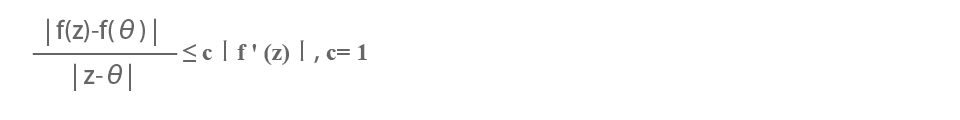This was posed in (Smale, 1981b) where it was proved for $c=4$. The constant $c$ has to be at least $\left(d-1\right)/d$ from the example $f\left(z\right)={z}^{d}-dz$. Tischler (1989) has some partial results.

Add.2 Is the three-sphere a minimal set?

Can a ${C}^{\infty }$ vector field be found on the three sphere so that every solution curve is dense?

I raised this problem in (Browder, 1976).

Add.3 Is an Anosov diffeomorphism of a compact manifold topologically the same as the Lie group model of John Franks?

This problem is in Franks' article in (Chern-Smale, 1970) where everything is made precise. Briefly an Anosov diffeomorphism is one where the tangent bunndle has a global invariant splitting into contracting and expanding subbundles (global hyperbolicity) and the non-wandering set is dense (see problems 10 and 11). It is not even known if the universal covering manifold must be Euclidean space. See also (Smale, 1967, 1980) for background.

References

Abraham,R. and Marsden,J., (1978). Foundations of Mechanics. Addison-Wesley Publishing Co., Reading, Mass.

Babin,A.V. and Vishik,M.I., (1983). Attractors of partial differential evolution equations and their dimension. Russian Math. Survey 38, 151-213.

Baker, A., (1979). Transcendental Number Theory, Cambridge University Press, Cambridge.

Barrow-Green, J., (1997). Poincaré and the Three Body Problem, American Math Society, Providence, RI.

Barvinok,A. and Vershik,A., (1993). Polynomial-time, computable approximation of families of semi-algebraic sets and combinatorial complexity. Amer. Math. Soc. Trans. 155, 1-17.

Bass,H., Connell,E., and Wright,D., (1982). The Jacobian conjecture: reduction on degree and formal expansion of the inverse. Bull. Amer. Math. Soc. 7, 287-330.

BCSS: Blum,L., Cucker,F., Shub,M., and Smale,S., (1997). Complexity and Real Computation, Springer-Verlag.

Blum,L., Shub,M. and Smale,S., (1989). On a theory of computation and complexity over the real numbers: NP-completness, recursive functions and universal machines. Bulletin of the Amer. Math. Soc. 21, 1-46.

Blum,L. and Smale,S., (1993). The Göodel incompleteness theorem and decidability over a ring. Pages 321{339 in M.Hirsch, J.Marsden and M.Shub (editors), From Topology to Computation: Proceedings of the Smalefest, Springer-Verlag.

Browder,F. ed., (1976). Mathematical Developments Arising from Hilbert Problems, American Math Society, Providence, RI.

Brownawell,W., (1987). Bounds for the degrees in the Nullstellensatz. Annals of Math. 126,577-591.

Chern,S. and Smale,S., eds. (1970). Proceedings of the Symposium on Pure Mathematics,vol. XIV, American Math Society, Providence, RI.

Chorin,A., Marsden,J., (1993). A Mathematical Introduction to Fluid Mechanics, 3rd edition,Springer-Verlag, New York.

Chorin,A., Marsden,J. and Smale,S., (1977). Turbulence Seminar, Berkeley 1976-77, LectureNotes in Math. 615, Springer-Verlag, New York.

Coomes, B., Kocak, H., and Palmer, K., (1996). Shadowing in discrete dynamical systems.in Six Lectures in Dynamical Systems, (eds. Aulbach, B. and Colonius, F.) World Scientific,Singapore.

Costa N. da and Doria, F., (1991). Undecidability and Incompleteness in Classical Mechanics. Internat. Jour. Theoretical Physics 30 1041{1073.

Cucker, F., M. Karpinski, M., Koiran, P., Lickteig, T., amd Werther, K., (1995). On real Turing machines that toss coins. In 27th annual ACM Symp. on the Theory of computing pp 335-342.

Cucker,F., Koiran,P., and Smale,S., (1997). A polynomial time algorithm for Diophantine equations in one variable. To appear, Jour. of Symbolic Computation.

Cucker, F. and Smale, S. (1997). Complexity estimates depending on condition and round-offerror. Preprint, to appear.

Debreu,G., (1959). Theory of Value, Wiley, New York.

Diacu, F. and Holmes, P., (1996). Celestial Encounters, Princeton University Press, Princeton,N. J.

Dulac,H., (1923). Sur les cycles limites. Bull. Soc. Math. France 51, 45-188.

Easton, R., (1971). Some topology of the 3 body problem. Jour. of Diff. Equations. 10,371-377.

Écalle,J., (1992). Introduction aux Fonctions Analysables et Preuve Constructive de la Conjecture de Dulac. Hermann, Paris.

van den Essen,A., (1997) Polynomial automorphisms and the Jacobian conjecture. in " Algèbre non commutative, groupes quantiques et invariants, septième contact Franco-Belge, Reims,Juin 1995". eds. J. ALev and G. Cauchon, "Société mathématique de France", Paris.

Freedman,M. (1982). The topology of 4-manifolds. J. Diff. Geom. 17, 357-454.

Garey,M. and Johnson,D., (1979). Computers and Intractability. Freeman, San Francisco.

Graczyk,J. and Swiatek,G., (1997). Generic hyperbolicity in the logistic family. Annals of Math. 146, 1-52.

Gr¨otschel,M., Lovasz,L., and Schrijver,A., (1993). Geometric Algorithms and Combinatorial Optimization, Springer-Verlag, New York.

Guckenheimer,J. and Holmes,P. (1990). Nonlinear Oscillations, Dynamical Systems and Bifurcations of Vector Fields, third printing, Springer-Verlag, New York.

Guckenheimer,J. and Williams,R.F. (1979). Structual stability of Lorenz attractors. Publ. Math. IHES 50, 59-72.

Hayashi,S., (1997). Connecting invariant manifolds and the solution of the C1 stability conjecture andstability conjecture for flows. Annals of Math. 145, 81-137.

Hirsch,M. and Smale,S., (1974). Differential Equations, Dynamical Systems, and Linear Algebra, Academic Press, New York.

Ilyashenko,Yu., (1985). Dulac's memoir "On limit cycles" and related problems of the local theory of di erential equations. Russian Math. Surveys VHO, 1-49.

Ilyashenko,Yu., (1991). Finiteness Theorems for Limit Cycles, American Math Society,Providence, RI.

Ilyashenko,Yu. and Yakovenko,S., (1995). Concerning the Hilbert 16th problem. AMS Translations, series 2, vol. 165, AMS, Providence, RI.

Jakobson,M., (1971). On smooth mappings of the circle onto itself. Math. USSR Sb. 14,161-185.

Kuijlaars,A.B.J. and Saff,E.B., (1997). Asymptotics for minimal discrete energy on the sphere. Trans. Amer. Math. Soc., to appear.

Lagarias, J., (1979). Succinct certificates for the solvability of binary quadratic diophantine equations, Proc. 20th IEEE Symposium on Foundations of Computer Science, Proc IEEE,47-54.

Lagarias, J., (1980). On the computational complexity of determining the solvability or unsolvability of the equation ${X}^{2}-D{Y}^{2}=-1$. Trans. Amer. Math. Soc. 260, 485-508.

Lang,S., (1991). Number Theory III, vol. 60 of Encyclopaedia of Mathematical Sciences,Springer-Verlag, New York.

Lenstra, H., (1997). Finding small degree factors of lacunary polynomials, preprint.

Linz,A., de Melo,W., and Pugh,C., (1977), in Geometry and Topology, Lecture Notes in Math. 597, Springer-Verlag, New York.

Liu,V., (1992). An example of instability for the Navier-Stokes equations on the 2-dimensional torus. Commun. PDE 17, 1995-2012.

Lloyd,N.G. and Lynch,S., (1988). Small amplitude limit cycles of certain Lienard systems. Proceedings Roy. Soc. London 418, 199-208.

Lorenz,E., (1963). Deterministic non-periodic flow. J. Atmosph. Sci. 20, 130-141.

Lyubich,M., (1997), Dynamics of Quadratic Polynomials. 1-2, Acta Mathematica 178, 185-297.

Manders,K.L. and Adleman,L., (1978). NP-complete decision problems for binary quadratics. J. Comput. System Sci. 16, 168-184.

Matiyasevich, Y., (1993). Hilbert's Tenth Problem. The MIT Press, Cambridge, Mass.

Mazur, B., (1994). Questions of decidability and undecidability in number theory. The Journal of Symbolic Logic 59, 353-371.

McCord, C., (1996). Planar central configuration estimates in the n-body problem. Ergodic Theory Dynamical Systems 5, 1059-1070.

McCord, C., Meyer, K., and Wang, Q., (1998) The Integral Manifolds of the Three Body Problem. to appear, Memoirs, Amer. Math. Soc., Providence,R.I.

McMullen,C., (1994). Frontiers in complex dynamics. Bull. Amer. Math. Soc. 31, 155-172.

de Melo,W. and van Strien,S., (1993). One-Dimensional Dynamics. Springer-Verlag, New York.

Palis,J. and Yoccoz,J.C., (1989). (1) Rigidity of centralizers of diffeomorphisms. Ann. Scient. Ecole Normale Sup. 22, 81-98; (2) Centralizer of Anosov diffeomorphisms. Ann.Scient. Ecole Normale Sup. 22, 99-108.

Palmore,J., (1976). Measure of degenerate relative equilibria, I. Annals of Math. 104,421-429.

Petrovskii,I.G. and Landis,E.M., (1957). On the number of limit cycles of the equation $dy/dx=P\left(x,y\right)/Q\left(x,y\right)$, where P and Q are polynomials. Mat. Sb. N.S. 43 (85), 149-168 (in Russian), and (1960) Amer. Math. Soc. Transl. (2) 14, 181-200.

Petrovskii,I.G. and Landis,E.M., (1959). Corrections to the articles \On the number of limit cycles of the equation $dy/dx=P\left(x,y\right)/Q\left(x,y\right)$, where P and Q are polynomials". Mat. Sb. N.S. 48 (90), 255{263 (in Russian)

Penrose,R. (1991). The Emperor's New Mind. Penguin Books.

Poincaré,H., (1953). Oeuvres, VI. Gauthier-Villars, Paris. Deuxième Complément à L'Analysis Situs-

Peixoto,M., (1962). Structural stability on two-dimensional manifolds. Topology 1, 101-120.

Pugh,C., (1967). An improved closing lemma and a general density theorem. Amer. J.Math. 89, 1010-1022.

Pugh,C. and Robinson,C., (1983). The ${C}^{1}$ closing lemma including Hamiltonians. Ergod.Theory Dynam. Systems 3, 261-313.

Rakhmanov,E.A., Saff,E.B. and Zhou,Y.M., (1994). Minimal discrete energy on the sphere.Math. Res. Lett. 1, 647-662.

Robinson,C., (1989). Homoclinic bifurcation to a transitive attractor of Lorenz type. Nonlineatity 2, 495-518.

Ruelle,D. and Takens,F., (1971). On the nature of turbulence. Commun. Math. Phys. 20,167-192.

Samuelson,P., (1971). Foundations of Economic Analysis, Atheneum, New York.

Saff,E. and Kuijlaars,A., (1997). Distributing many points on a sphere. Math Intelligencer 10, 5-11.

Schrijver,A., (1986). Theory of Linear and Integer Programming. John Wiley & Sons.

Shi,S., (1982). On limit cycles of plane quadratic systems. Sci. Sin. 25, 41-50.

Shub,M., (1970). Appendix to Smale's paper: Diagonals and relative equilibria in manifolds,Amsterdam, 1970. Lecture Notes in Math. 197, Springer-Verlag, New York.

Shub,M. and Smale,S., (1995). On the intractibility of Hilbert's Nullstellensatz and an algebraic version of "$P=NP$", Duke Math. J. 81, 47-54.

Shub,M. and Smale,S., (1993). Complexity of Bezout's theorem, III: condition number and packing. J. of Complexity 9, 4{14.

Shub,M. and Smale,S., (1994). Complexity of Bezout's theorem, V: polynomial time. The-oret. Comp. Sci. 133, 141-164.

Smale,S., (1963). Dynamical systems and the topological conjugacy problem for diffeomorphisms, pages 490-496 in: Proceedings of the International Congress of Mathematicians,Inst. , Sweden, 1962. (V.Stenström, ed.)

Smale,S., (1963). A survey of some recent developments in di erential topology. Bull. Amer.Math. Soc. 69, 131-146.

Smale,S., (1967). Di erentiable dynamical systems. Bull. Amer. Math. Soc. 73, 747-817.

Smale,S., (1970). Topology and mechanics, I and II. Invent. Math. 10, 305-331 and Invent.Math. 11, 45-64.

Smale,S., (1976). Dynamics in general equilibrium theory. Amer. Economic Review 66,288-294.

Smale,S., (1980). Mathematics of Time, Springer-Verlag, New York.

Smale,S., (1981a). Global analysis and economics, pages 331-370 in Handbook of Mathematical Economics 1, editors K.J.Arrow and M.D.Intrilligator. North-Holland, Amsterdam.

Smale, S. (1981b). The fundamental theorem of algebra and complexity theory. Bulletin of the Amer. Math. Soc. 4, 1-36.

Smale,S., (1990a). The story of the higher dimensional Poincaré conjecture. Mathematical Intelligencer 12, no. 2, 40-51. Also in M.Hirsch, J.Marsden and M.Shub, editors, From Topology to Computation: Proceedings of the Smalefest, 281{301 (1992).

Smale, S., (1990b). Some remarks on the foundations of numerical analysis. SIAM review 32, 211-220.

Smale,S., (1991). Dynamics retrospective: great problems, attempts that failed. Physica D 51, 267-273.

Smale, S., (1998). Finding a horseshoe on the beaches of Rio, The Mathematical Intelligencer 20, 39-44.

Taubes,G., (July 1987). What happens when Hubris meets Nemesis? Discover.

Temam,R., (1979). Navier-Stokes Equations, revised edition. North-Holland, Amsterdam.

Tischler, D., (1989). Critical points and values of complex polynomials. Jour. of Complexity 5, 438-456.

Traub,J. and Wozniakowski,H., (1982). Complexity of linear programming. Oper. Res.Letts. 1, 59-62.

Tsuji,M., (1959). Potential Theory in Modern Function Theory. Maruzen Co., Ltd., Tokyo.

Wen,L. and Xia,Z., (1997). A simpler proof of the C1 connecting lemma. To appear, Trans.of the Amer. Math. Soc.

Williams,R., (1979). The structure of Lorenz attractors. Publ. IHES 50, 101-152.

Wintner,A., (1941). The Analytical Foundations of Celestial Mechanics. Princeton University Press, Princeton, NJ.